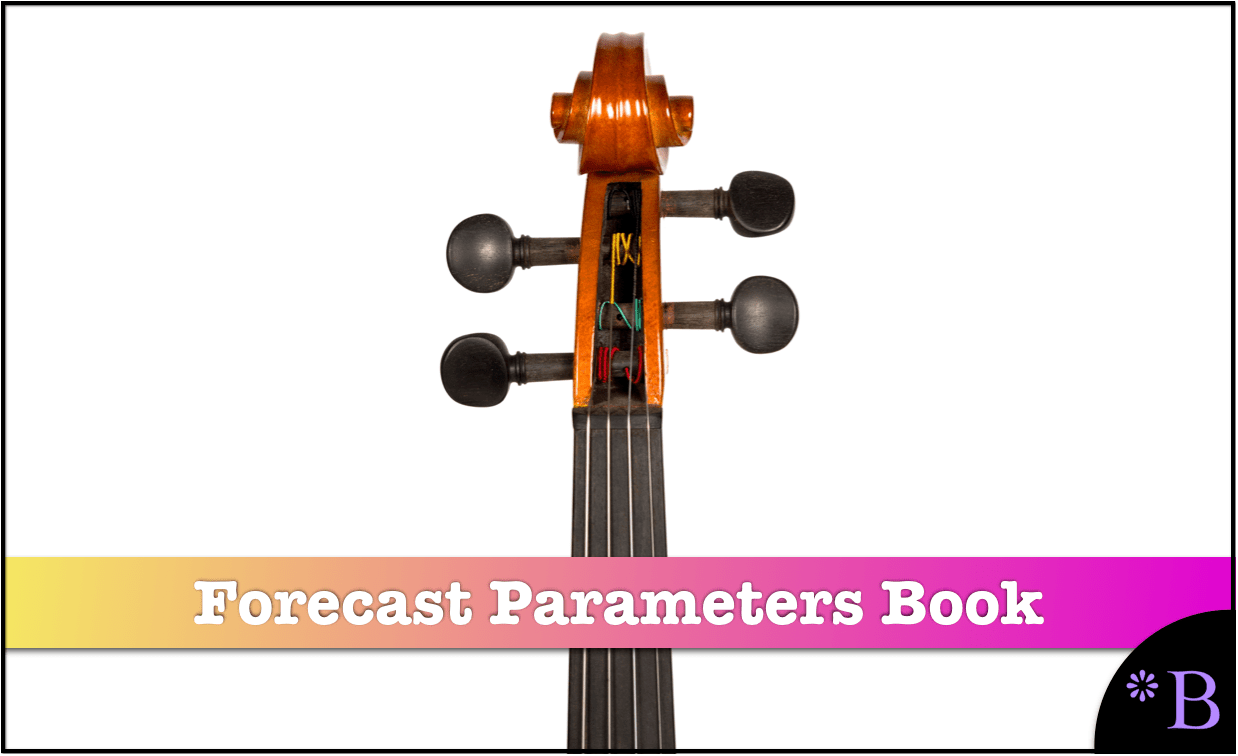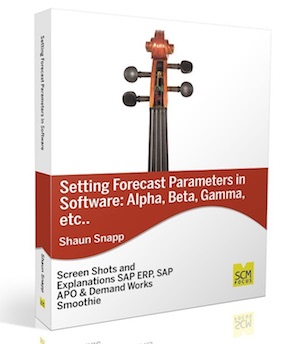#Setting Forecast Parameters in Software: Alpha Beta Gamma, etc

Screenshots and examples from SAP ERP, SAP APO, Demand Works Smoothie.

## What The Book Covers

Finally, a book that focuses in-depth on forecast parameters like alpha beta gamma as well as others.

Forecast parameters are one of the least understood areas of statistical forecasting systems, and statistical forecasting is used as a vast number of areas. This is the first book to focus exclusively on this arcane topic.

This book takes up the topic of statistical forecast parameters as a way to make improvements in the forecast models that drive statistical forecast accuracy.

By reading this book, you will:

• See how forecast parameters like alpha beta gamma and others are set in 3 different well-known statistical forecasting applications.
• Learn from real-world projects how forecast parameters are tuned and the knowledge transferred to the forecast planners.
• Understand the importance of being able to evaluate and review the forecast parameters externally.
• Understand the importance of the assignment between the resulting Forecast Profiles (that are the combination of a forecast model with parameters) to the forecasted items.
• Learn how some forecasting applications allow direct manipulation of forecast parameters like alpha beta gamma and others and how other forecasting applications internally determine the parameters.
• Learn about specific statistical forecasting models that use no externally determined parameters regardless of the application used.

## A Book Based in Reality

The book provides many examples from real-life project experiences, the emphasis being on the reality of planning projects.

## Interconnected to Web Information

In order the keep the book at a manageable and easily readable length, the book also provides numerous links out to the Brightwork Research & Analysis site, where supporting articles allow readers to get into more detail on topics that interest them.

# Chapters

• Chapter 1: Introduction
• Chapter 2: Where Forecasting Fits in the Supply Chain Footprint
• Chapter 3: Statistical Forecasting Explained
• Chapter 4: Forecast Parameters
• Chapter 5: Introduction to Best Fit Forecasting
• Chapter 6: Conclusion

## Chapter 1: Introduction

• Important Terminology: The Approach to the Book

## Chapter 2: Where Forecasting Fits in the Supply Chain Planning Footprint

• Background
• Where Demand Planning Software within Supply Chain Software
• The Demand Planning Application Categories
• The Forecast Category, Method and Model Employed
• Connecting the Demand Planning System to Supply Planning
• Conclusion

## Chapter 3: Statistical Forecasting Explained

• The Initial Attempts at Demand Planning Software Implementation
• What Makes Statistical Forecasting “Statistical”?
• Statistical Forecasting Versus Other Forms of Statistics Such as Polling
• Statistical Forecasting and Inferential Statistics
• The Relationship between Probability Theory and Statistics
• Statistical Forecasting Methods
• Time Series Techniques
• Exponential Smoothing
• Regression or Causal Forecasting
• The Actual use of Regression or Causal Forecasting
• Correlation Versus Causation
• High-Level Causal Models
• Implementing Causal Forecasting
• Best-Fit Functionality
• The General Confusion on Best Fit Functionality
• Best-Fit Functionality and the Selection of the Constant Model
• Best-Fit Statistics
• Manual Adjustments to the Statistical Forecast
• User Adoption Issues and the Type of Forecasting System Selected
• Conclusion
• Case Study: Forecast Parameter Management
• Case Study: How Sales Teams Often Circumvent Client User Feedback

## Chapter 4: Forecasting Parameters

• System Default Values for Alpha, Beta, and Gamma
• Forecast Ranges for the Core Forecast Parameters
• The Secondary Forecast Parameters Definitions and Examples
• Time-Based Forecast Parameters: Definitions and Examples
• Default Values for Forecast Parameters
• Documenting Forecast Parameters per Forecast Profile
• MLR Profile
• Where Forecasting Parameters are Set
• Creating Specialized Forecasting Profiles
• Using Parameters
• Linear Regression – The Parameter-less Statistical Forecasting Model 40 Forecast Parameters in SAP ERP
• Conclusion

## Chapter 5: Introduction to Best Fit Forecasting

• Overestimating Best Fit Forecasting
• Common Problems with Best Fit Forecasting Functionality
• Best Fit and Overfitting
• Adjusting the Best Fit Forecast
• Conclusion

## Conclusion